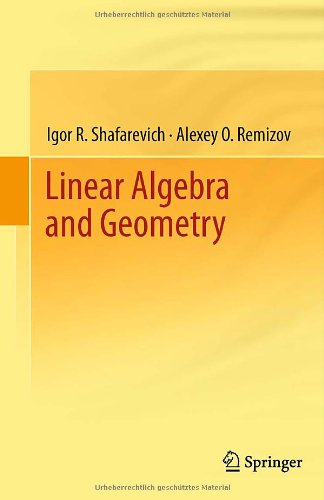# Linear Algebra and Geometry

• Length: 547 pages
• Edition: 2013
• Publisher:
• Publication Date: 2012-08-24
• ISBN-10: 3642309933
• ISBN-13: 9783642309939
Description

This book on linear algebra and geometry is based on a course given by renowned academician I.R. Shafarevich at Moscow State University. The book begins with the theory of linear algebraic equations and the basic elements of matrix theory and continues with vector spaces, linear transformations, inner product spaces, and the theory of affine and projective spaces. The book also includes some subjects that are naturally related to linear algebra but are usually not covered in such courses: exterior algebras, non-Euclidean geometry, topological properties of projective spaces, theory of quadrics (in affine and projective spaces), decomposition of finite abelian groups, and finitely generated periodic modules (similar to Jordan normal forms of linear operators). Mathematical reasoning, theorems, and concepts are illustrated with numerous examples from various fields of mathematics, including differential equations and differential geometry, as well as from mechanics and physics.